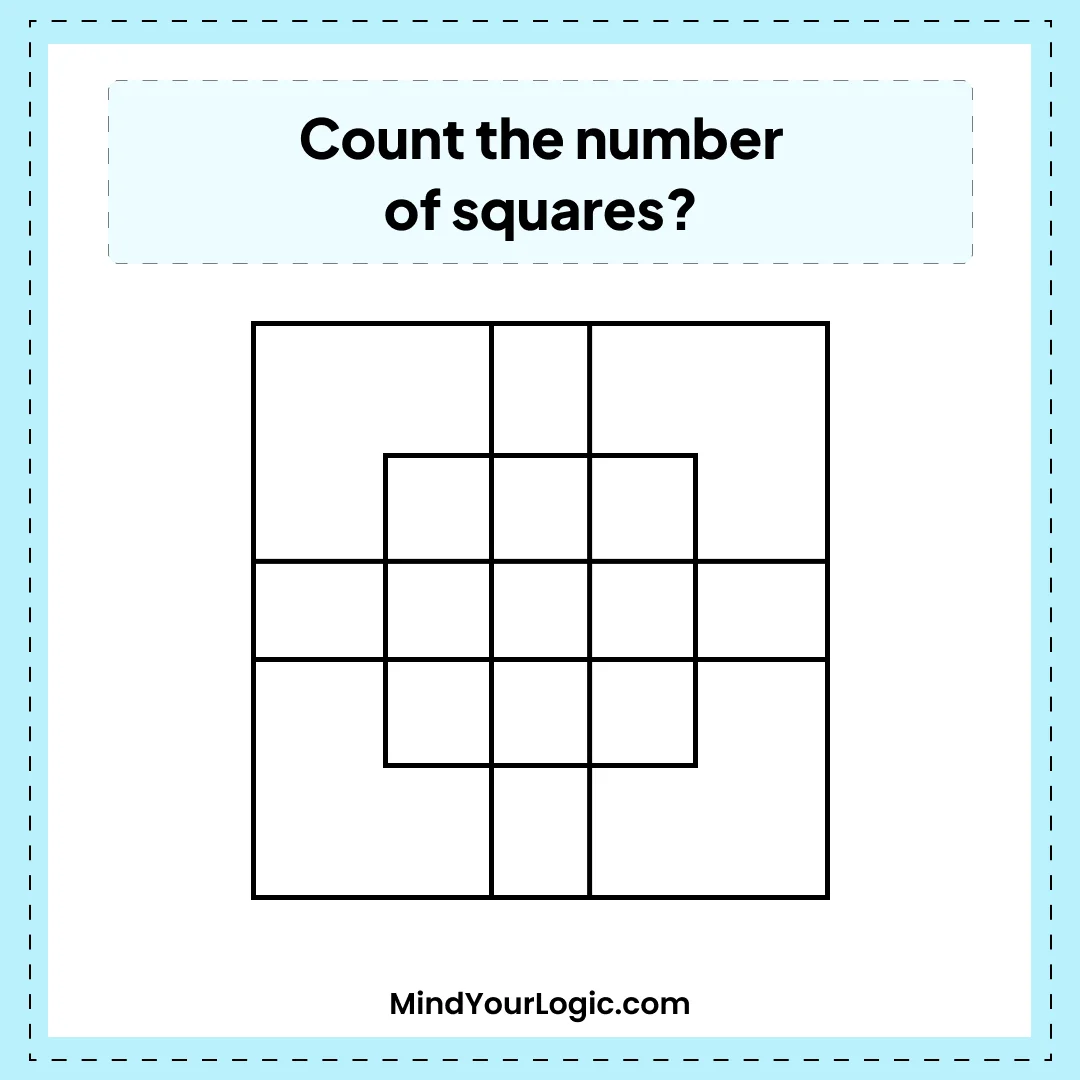# Count the number of squares - Math riddle

###### 16.Math Riddles
`Count the number of squares in the given figure?`• A.27
• B.23
• C.24
• D.31
• ```Explanation :

27
The simplest squares are EFRQ, MQYX, QRZY, RNSZ, LXWK, XY, A1W,
Y Z B1A1, ZSTB1, SGHT, W A1V P, A1B1UV, B1TOU and VUIJ  i.e. 13 in number
The squares having two components each are AEYL, FBGZ, KA1JD and B1HCI  i.e. 4 in number.
The squares having four components  each are MRB1W, QNTA1, XZUP and YSOV  i.e. 4 in number.
The squares having seven components each are AF1k, EBHA1, LZID and YGCJ  i.e. 4 in number.
There is only one square i.e. MNOP composed of nine components.
There is only one square i.e. ABCD composed of seventeen components.
There are 13+4+4+4+1+1 = 27 squares in the figure.```